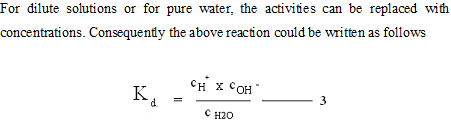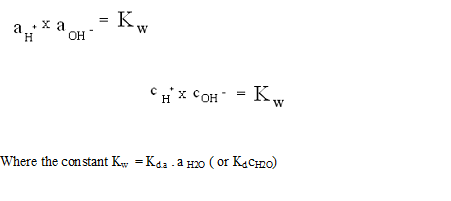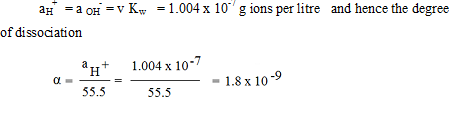## Lesson 17. pH AND pOH SCALES

Module 7. Electrical conductivity

Lesson 17
pH AND pOH SCALES

17.1 Introduction

When water molecule dissociates and attains ionic equilibrium the concentration of both [H+] and [OH-] ions will be equal. Increase of either H+ or OH- would result in the imbalance of the equation. As such this phenomenon is used to measure the acidic or alkaline condition of the solution. This is referred as pH scale. Due to the large range of proton concentration values [H+] in aqueous solution (typically from 10-15 to 10 M), a logarithmic scale of acidity makes the most sense to put the values into manageable numbers.

17.2 Definition

The pH is a quantity characterizing the activity (or concentration) of hydrogen ions and is numerically equal to the negative common logarithm of the activity or concentration expressed in g-ions/l
The pH scale of acidity defines pH as the negative common logarithm of the concentration of H+: pH = - log [H+]
A common logarithm is a function that computes what exponent would be on 10 to obtain the input number. For example, the logarithm of 100 (written "log 100") is 2 because 102 = 100
Although water is frequently regarded as a substance that practically does not dissociate into ions it is in fact always dissociated to a small extent according to the following equation

H2O ↔ H+ + OH- ---------- 1

In solutions containing acids the hydrogen ions form will affect the equilibrium of the above reaction. Similarly in solutions containing bases the hydroxyl ions will affect the equilibrium. The equilibrium is determined by the dissociationIn the water dissociation reaction, equilibrium greatly shifts in the direction of undissociated water, but it is very readily established and this imparts considerable importance to the role played by the reaction (1) in many properties of aqueous solutions. Since the dissociation of water being very slight, the activity or concentration of undissociated water molecule is regarded as constant and combining this constant with the dissociation constant we write the following equations.This constant is called the ionic product of water. At 250°C
the ionic product of water.

Kw = 1.008 x 10-14
since in pure water as in any other neutral medium
aH+= aOH-or(cH+ = cOH- ) for 25
°C
­­­­­­­­­­­­­­­­­Where 55.5 = CH2O i.e the number of moles of water per liter at 25°C.

In non neutral media, the activities aH+ and an OH- are not equal to each other. And they are inversely proportional to each other. Thus on adding the acid to water we increase the concentration of hydrogen ions and therefore augment the value of aH+ but this will accelerate the opposite direction of reaction. Some of the added hydrogen ions bind an equivalent amount of OH- to form H2O and a reduction in a OH- occurs. Equilibrium is established again when the product of the ion activities once more acquires the value it had prior to the addition of the acid. Hence any increase in hydrogen ion concentration causes a corresponding decrease in hydroxyl ion concentration and vice versa.

The simple reaction between these two quantities may be used to characterize the acidity and alkalinity of media. The acid properties are due to the hydrogen ion and can be expressed quantitatively by the activity of aH+. Similarly the alkalinity of a medium can be expressed by the activity of a OH-. Owing to the single valued relation between these two only one of them can be used to characterize both acidity and alkalinity. Although both are equivalent in this respect it is customary to use the activity of the hydrogen ions as the parameter utilized by convention.

In acid solution aH+ is greater than in pure water, the difference increasing as the acidity increases. In alkaline media the Value of aH+ is less than for pure water again the difference increasing as the alkalinity increases. In practice instead of aH+ the so called hydrogen ion exponent of pH is used which is defined as

pH = -log10 aH+

For a dilute solutions pH = -log10 cH+

Hence pH is a quantity characterizing the activity (or concentration) of hydrogen ion and is numerically equal to the negative common logarithm of the activity of concentration expressed as g- ions per litre.

At 25°C the pH of a neutral medium is 7, acidic solution has a pH value less than 7 because [H+] is greater than that of pure water. This is known as neutral pH. This value is not randomly selected, but rather it comes from the fact that the [H+] in pure water is 10-7 (recall that Kw = 10-14). A basic solution has a pH greater than 7 because there is a lower [H+] than that of pure water (and consequently a larger OH]).

Chemists use a pOH scale analogous to the pH acidity scale to gauge hydroxide ion concentrations of aqueous solutions. pOH is defined as the negative common logarithm of the concentration of OH-: pOH = -log [OH- ]. In the pOH scale, 7 is neutral, less than 7 is basic, (acidic) and greater than 7 is (basic) acidic. A useful relationship (which you should be able to derive using the definition of Kw) is that pH+ pOH = 14. This formula will allow you to readily convert values of pH and pOH. A comparison of the pH and pOH scales is provided in Fig. 17.1. Note that because Kw is constant, the product of [H+] and [OH-] is always equal to 10-14.Fig. 17.1 Comparison of the pH and pOH of acidity
(Source: Text book of Dairy Chemistry, Mathur et. al., 2005)

The prefix "p" in front of a symbol means "take the negative log". We have defined pH and pOH as values to describe the acidic or basic strength of solutions. Now we can talk about pKa (-log Ka) and pKb (-log Kb) as measures of acidity and basicity, respectively, for standard acids and bases. An acid with a pKa less than zero is called a strong acid because it almost completely dissociates in water, giving an aqueous solution a relatively low pH. Acids with pKa's greater than zero are called weak acids because they only partially dissociate in water, to make solutions with larger pH's than those strong acids produce at the same concentration. Similarly, bases with pKb's less than zero are strong bases and bases with pKb's greater than zero are called weak bases.